Polar animal with a very long tooth ! (8 - 10 years old)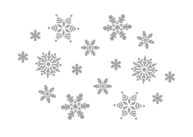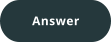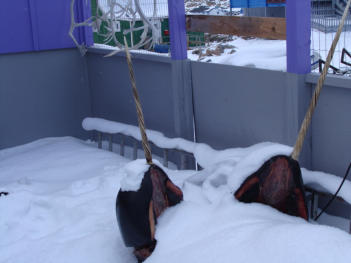Solve these calculations. An intruder is hidden in each of the 7 lines. Find them and read the corresponding letters. They are in the right order. So you will know the name of this polar animal which lives in the sea and has a very long tooth !
On the picture : heads brought back by an inuit fisherman!
1)
E = 450 : 6                       N = 987 - 362                        T = 1/2  x  150                       I = 15  x  5
2)
K = 1/10  x  100                Y = 90 - 80                             A = 2 x 2 x 5                          G = 100 : 10
3)
R = 750 : 5

H = 25 x 5                              Q = 1000 : 8                          U = 250 : 2
4)
B = 51 : 3                          O = 9 + 8                                P = 1/2  x  34                         V = 34 - 18
5)
S = 50 + 28                       A = 9 x 8                                Z = 120 - 42                           D = 1/3  x  234
6)
C = 9 x 6                            J =
102 - 48                          L = 27 x 3                              M = 2 x 3 x 3 x 3Polar animal with a very long tooth ! (8 - 10 years old)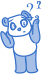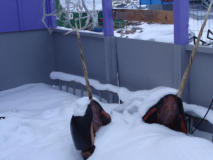Solve these calculations. An intruder is hidden in each of the 7 lines. Find them and read the corresponding letters. They are in the right order. So you will know the name of this polar animal which lives in the sea and has a very long tooth !
On the picture : heads brought back by an inuit fisherman!
1)
E = 450 : 6                       N = 987 - 362                        T = 1/2  x  150                       I = 15  x  5
2)
K = 1/10  x  100                Y = 90 - 80                             A = 2 x 2 x 5                          G = 100 : 10
3)
R = 750 : 5

H = 25 x 5                              Q = 1000 : 8                          U = 250 : 2
4)
B = 51 : 3                          O = 9 + 8                                P = 1/2  x  34                         V = 34 - 18
5)
S = 50 + 28                       A = 9 x 8                                Z = 120 - 42                           D = 1/3  x  234
6)
C = 9 x 6                            J =
102 - 48                          L = 27 x 3                              M = 2 x 3 x 3 x 3Polar animal with a very long tooth ! (8 - 10 years old)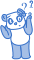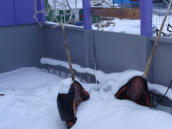Solve these calculations. An intruder is hidden in each of the 7 lines. Find them and read the corresponding letters. They are in the right order. So you will know the name of this polar animal which lives in the sea and has a very long tooth !
On the picture : heads brought back by an inuit fisherman!
1)
E = 450 : 6                       N = 987 - 362                        T = 1/2  x  150                       I = 15  x  5
2)
K = 1/10  x  100                Y = 90 - 80                             A = 2 x 2 x 5                          G = 100 : 10
3)
R = 750 : 5

H = 25 x 5                              Q = 1000 : 8                          U = 250 : 2
4)
B = 51 : 3                          O = 9 + 8                                P = 1/2  x  34                         V = 34 - 18
5)
S = 50 + 28                       A = 9 x 8                                Z = 120 - 42                           D = 1/3  x  234
6)
C = 9 x 6                            J =
102 - 48                          L = 27 x 3                              M = 2 x 3 x 3 x 3